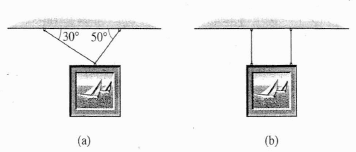# Tension and equilibrium

## Homework Statement

you want to hang a 15-N picture as in part (a) using some very fine twine that will break with more than 12 N of tension can you do this? what if you have it as illustrated in part (b) of the figure?Σf=ma

## The Attempt at a Solution

[/B]
okay i started with finding the tensions in part (a). using:
Σfx= 0 and so
T2 cos30=T1 cos50
T2=0.74 T1 ---------(1)
Σfy=0
T1sin50 + T2sin30= W
0.76T1+0.5T2 = 15-------(2)

substituting (1) in (2)

0.76T1 +0.5 (0.74 T1)=15
0.76 T1 + 0.37 T1 =15
1.13 T1= 15

T1= 13.27N
and so T2= 9.82 N

Now what i am not sure about, is first how to know if the ceiling is fine with these tensions or not? I mean should I only find the vertical forces of the tensions here (T1sin50 & T2 sin 30) and see if they are larger than 12 ( obviously they are ) or what?

and regarding the part B. I am also hesitated about using the same Tension values computed from the first part (a) in b also!

Last edited:

ehild
Homework Helper

## Homework Statement

you want to hang a 15-N picture as in part (a) using some very fine twine that will break with more than 12 N of tension can you do this? what if you have it as illustrated in part (b) of the figure?
View attachment 231773

Σf=ma

## The Attempt at a Solution

[/B]
okay i started with finding the tensions in part (a). using:
Σfx= 0 and so
T2 cos30=T1 cos50
T2=0.74 T1 ---------(1)
Σfy=0
T1sin50 + T2sin30= W
0.76T1+0.5T2 = 15-------(2)

substituting (1) in (2)

0.76T1 +0.5 (0.74 T1)=15
0.76 T1 + 0.37 T1 =15
1.13 T1= 15

T1= 13.27N
and so T2= 9.82 N

Now what i am not sure about, is first how to know if the ceiling is fine with these tensions or not? I mean should I only find the vertical forces of the tensions here (T1sin50 & T2 sin 30) and see if they are larger than 12 ( obviously they are not) or what?

and regarding the part B. I am also hesitated about using the same Tension values computed from the first part (a) in b also!

The string can withstand 12 N tension at maximum. You got more than 13 N tension for one of the strings. What would happen to it?
Case b is entirely different, the tensions are different, too.

•SakuRERE
ou got more than 13 N tension for one of the strings
okay, i got what you said, but the point that i want to ask still somehow not clear.
for example if i got for T2=5 N and for T1= 8N, and the ceiling is fine with 12 maximum.
do i say
T2 + T1= 5+8= 13 so no! it's more than the limit
or do i take only vertical components of the tensions like saying:
T2 cos 30 + T1 cos 50 =?
5 cos30 + 8 cos50 = 9.47 so the ceiling is fine with it?
Thanks

ehild
Homework Helper
okay, i got what you said, but the point that i want to ask still somehow not clear.
for example if i got for T2=5 N and for T1= 8N, and the ceiling is fine with 12 maximum.
do i say
T2 + T1= 5+8= 13 so no! it's more than the limit
or do i take only vertical components of the tensions like saying:
T2 cos 30 + T1 cos 50 =?
5 cos30 + 8 cos50 = 9.47 so the ceiling is fine with it?
Thanks
It is not the ceiling that is critical. The problem days "using some very fine twine that will break with more than 12 N of tension can you do this? " The twine will brake if overloaded! The tension in any of them can not exceed 12 N.
If the problem said that the ceiling can not withstand more than 12 N load, you might use the sum of the vertical components of the tensions.